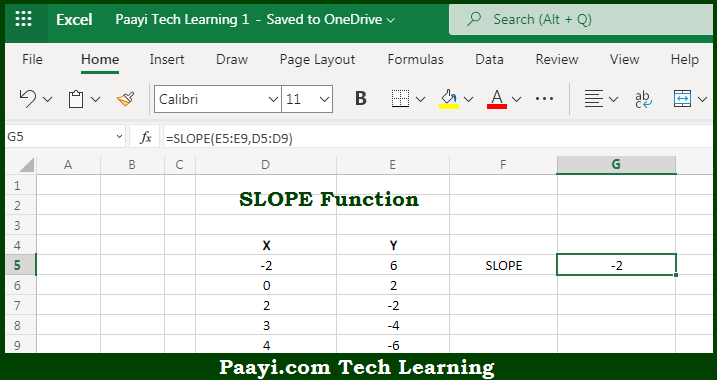# Learn How to Use Microsoft Excel SLOPE Function

Written by | 0 Comments | 499 Views

In this article, you will learn how to use the Microsoft Excel SLOPE function and its prime function in Microsoft Excel. You will also get to know the Microsoft Excel SLOPE function return value and syntax with the help of some examples.

Microsoft Excel SLOPE Function

The main purpose of the Microsoft Excel SLOPE function is to get the slope of linear regression line. That implies, with the help of SLOPE function you can able to return the slope of a regression line based on known "y" values and known "x" values. It should be noted that the regression line is a "best fit" line based on known data points. So, with the help of the SLOPE function, you can able to get the slope of the linear regression line.

Return Value of SLOPE Function

The return value will be the calculated slope of the number.

Syntax of SLOPE Function

=SLOPE(known-ys, known-xs)

Where the arguments:

• known-ys: This is the range or array of numeric data points which are dependent values.
• known-xs: This is the range or array of numeric data points which are independent values.

## How to Use Microsoft Excel SLOPE Function?So we know that Microsoft Excel SLOPE function you can able to get the slope of a linear regression line. That implies, with the help of SLOPE function you can able to return the slope of a regression line based on known "y" values and known "x" values. It should be noted that the regression line is a "best fit" line based on known data points. So, with the help of the SLOPE function, you can able to get the slope of a linear regression line.